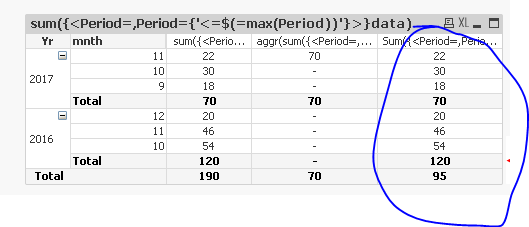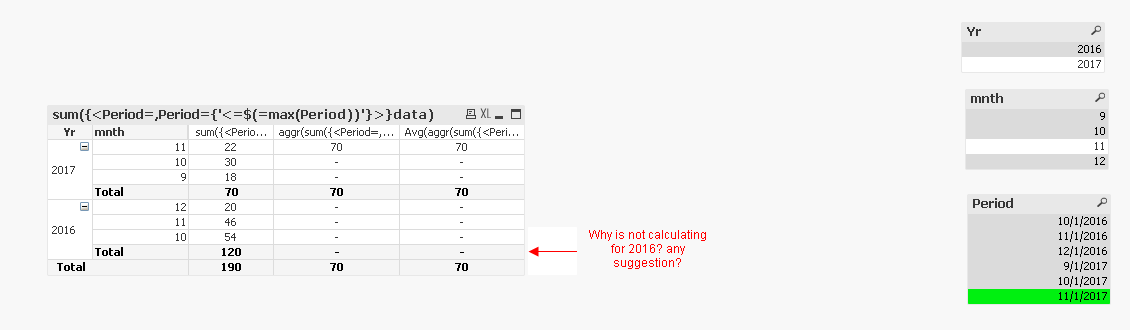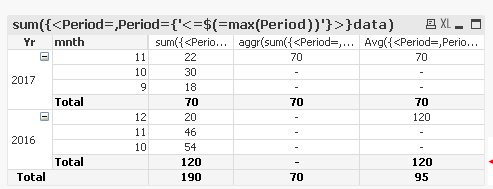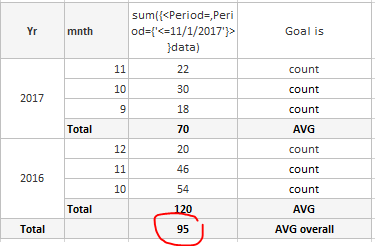# QlikView App Dev

Discussion Board for collaboration related to QlikView App Development.

Announcements
Join us at the Cloud Data and Analytics Tour! REGISTER TODAY
cancel
Showing results for
Search instead for
Did you mean:Creator

## line level count and avg at total is it possible?

Hi All

is it possible to count by month and Total would be Average?1 Solution

Accepted SolutionsMVP

This?

Sum({<Period=,Period={'<=\$(=max(Period))'}>}aggr(sum({<Period=,Period={'<=\$(=max(Period))'}>}data),Yr, mnth))/Count({<Period=,Period={'<=\$(=max(Period))'}>}DISTINCT Yr)11 RepliesMVP

Sure... it would be something like this may be

Avg(Aggr(Count(Measure), SnapYear, SnapMonth))Creator
Author

It didnt work

AVG(aggr(Count( {<[Year/Month],Period={'<=\$(=max(Period))'}>} EMPLID),YEAR,MONTH))

with this I got was only Oct (As user has selected Oct) no other months showed

2017    10      122878

I dont know what that 122878 number is..not the AVG...I have all values below 80000Champion III

Can you share a sample app to look into?MVP

First of all, let me ask you this... did it not work at all, or did it not work as your expected? The reason it did not work as expected it because you failed to provide complete information.... do you expect me to know your expression and give you a result that would work for you? I am sorry, but you will have a hard time all the time...

Anyways, try adding the set analysis from your inner aggregation to the outer one and see if this resolves your problem.

Avg({<[Year/Month],Period={'<=\$(=max(Period))'}>}Aggr(Count( {<[Year/Month],Period={'<=\$(=max(Period))'}>} EMPLID),YEAR,MONTH))Creator
Author

Sunny, Thanks for help. Much appreciated.

Still AGGR() and AVG() producing some results difficult to figure out what they are doing. I have to dig more on these functions. I feel in QV these 2 functions are not straight forward.MVP

So the value for the row level isn't the right number? or just the totals are off?Creator
Author

Attached is an app I was able to reproduce problem in sample data. Any suggestions why it is not calculating roll up for 2016 and work around?

ThanksMVP

Is this the goal?Used this

Avg({<Period=,Period={'<=\$(=max(Period))'}>}aggr(sum({<Period=,Period={'<=\$(=max(Period))'}>}data),Yr))Creator
Author

Desired state is where total for year would be AVG, right now with this change in statement we have sum as total for year and overall is AVG but in separate column. If I get Goal is shown below is Ideal but even if I have 70/120 sum as totals and 95 (AVG) as overall total I would be happy and not spending time any further.